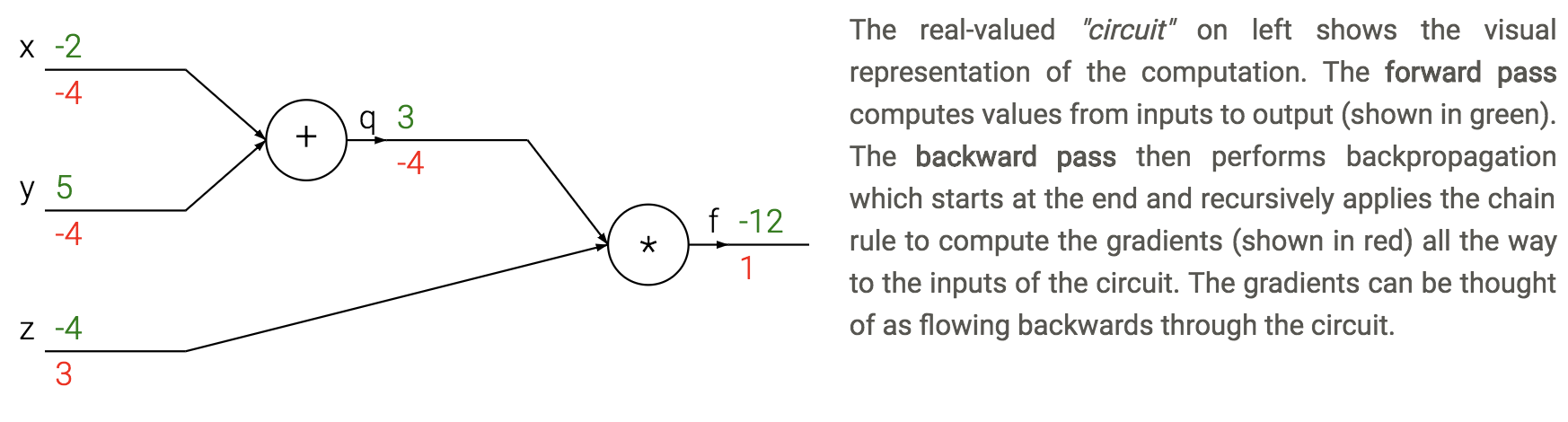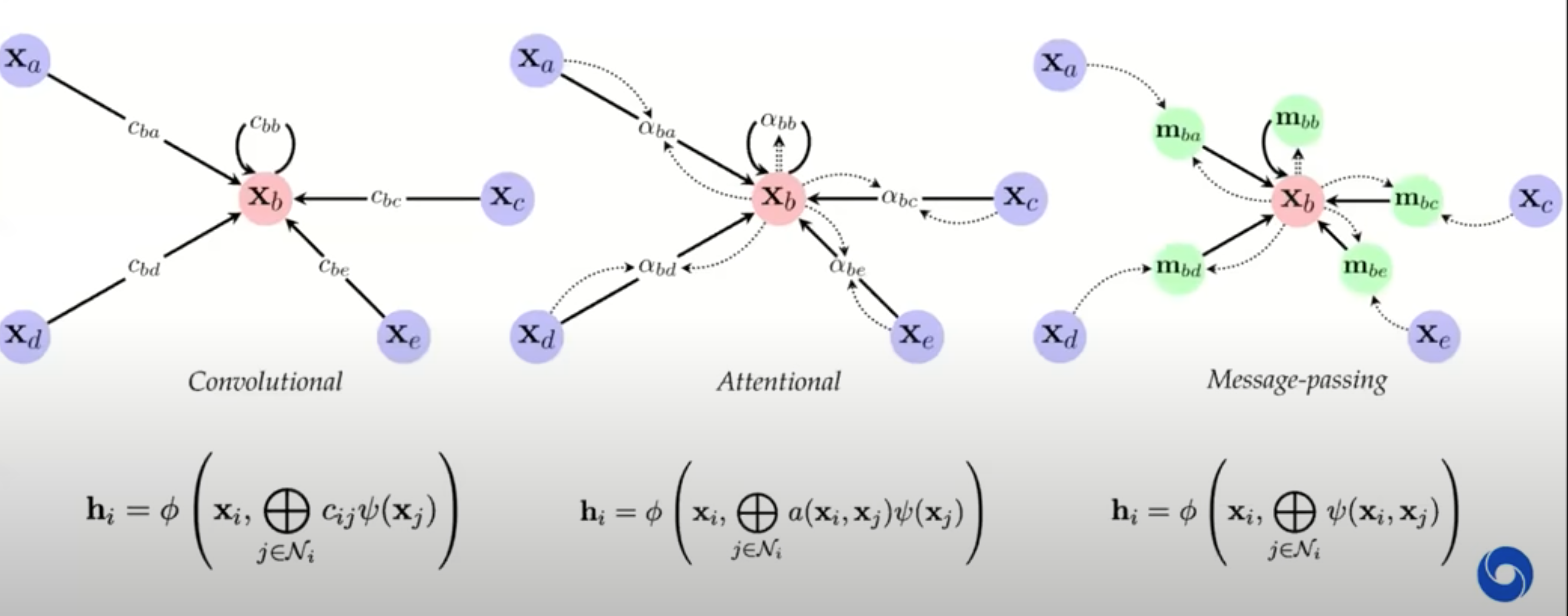deep learning

This note covers miscellaneous deep learning, with an emphasis on different architectures + empirical tricks.

See also notes in 📌 unsupervised learning, 📌 disentanglement, 📌 nlp, 📌 transformers

# top-performing nets

• LeNet (1998)
• first, used on MNIST
• AlexNet (2012)
• landmark (5 conv layers, some pooling/dropout)
• ZFNet (2013)
• fine tuning and deconvnet
• VGGNet (2014)
• 19 layers, all 3x3 conv layers and 2x2 maxpooling
• lots of parallel elements (called Inception module)
• Msft ResNet (2015)
• very deep - 152 layers
• connections straight from initial layers to end
• only learn “residual” from top to bottom
• Region Based CNNs (R-CNN - 2013, Fast R-CNN - 2015, Faster R-CNN - 2015)
• object detection
• Generating image descriptions (Karpathy , 2014)
• RNN+CNN
• Spatial transformer networks (2015)
• transformations within the network
• Segnet (2015)
• encoder-decoder network
• Unet (Ronneberger, 2015)
• applies to biomedical segmentation
• Pixelnet (2017) - predicts pixel-level for different tasks with the same architecture - convolutional layers then 3 FC layers which use outputs from all convolutional layers together
• Squeezenet
• Yolonet
• Wavenet
• Densenet
• NASNET
• Efficientnet (2019)

# basics

• basic perceptron update rule
• if output is 0, label is 1: increase active weights
• if output is 1, label is 0: decrease active weights
• perceptron convergence thm - if data is linearly separable, perceptron learning algorithm wiil converge
• transfer / activation functions
• sigmoid(z) = $\frac{1}{1+e^{-z}}$
• Binary step
• TanH (preferred to sigmoid)
• Rectifier = ReLU
• Leaky ReLU - still has some negative slope when <0
• rectifying in electronics converts analog -> digital
• rare to mix and match neuron types
• deep - more than 1 hidden layer
• regression loss = $\frac{1}{2}(y-\hat{y})^2$
• classification loss = $-y \log (\hat{y}) - (1-y) \log(1-\hat{y})$
• can’t use SSE because not convex here
• multiclass classification loss $=-\sum_j y_j \ln \hat{y}_j$
• backpropagation - application of reverse mode automatic differentiation to neural networks’s loss
• apply the chain rule from the end of the program back towards the beginning
• $\frac{dL}{d \theta_i} = \frac{dL}{dz} \frac{\partial z}{\partial \theta_i}$
• sum $\frac{dL}{dz}$ if neuron has multiple outputs z
• L is output
• $\frac{\partial z}{\partial \theta_i}$ is actually a Jacobian (deriv each $z_i$ wrt each $\theta_i$ - these are vectors)
• each gate usually has some sparsity structure so you don’t compute whole Jacobian
• pipeline
• initialize weights, and final derivative ($\frac{dL}{dL}=1$)
• for each batch
• run network forward to compute outputs at each step
• compute gradients at each gate with backprop
• update weights with SGD
•# training

• happens with sigmoids
• exploding gradients problem - gradients are significantly larger in earlier layers than later layers
• RNNs
• batch normalization - whiten inputs to all neurons (zero mean, variance of 1)
• do this for each input to the next layer
• dropout - randomly zero outputs of p fraction of the neurons during training
• like learning large ensemble of models that share weights
• 2 ways to compensate (pick one)
1. at test time multiply all neurons’ outputs by p
2. during training divide all neurons’ outputs by p
• softmax - takes vector z and returns vector of the same length
• makes it so output sums to 1 (like probabilities of classes)
• tricks to squeeze out performance
• ensemble models
• (stochastic) weight averaging can help a lot
• test-time augmentation
• this could just be averaging over dropout resamples as well
• 10x larger DNNs into memory with 20% increase in comp. time
• save gradients for a carefully chosen layer to let you easily recompute

# CNNs

• kernel here means filter
• convolution G- takes a windowed average of an image F with a filter H where the filter is flipped horizontally and vertically before being applied
• G = H $\ast$ F
• if we do a filter with just a 1 in the middle, we get the exact same image
• you can basically always pad with zeros as long as you keep 1 in middle
• can use these to detect edges with small convolutions
• can do Guassian filters
• 1st-layer convolution typically sum over all color channels
• 1x1 conv - still convolves over channels
• pooling - usually max - doesn’t pool over depth
• people trying to move away from this - larger strides in conversation layers
• stacking small layers is generally better
• most of memory impact is usually from activations from each layer kept around for backdrop
• visualizations
• layer activations (maybe average over channels)
• visualize the weights (maybe average over channels)v
• feed a bunch of images and keep track of which activate a neuron most
• t-SNE embedding of images
• occluding
• weight matrices have special structure (Toeplitz or block Toeplitz)
• input layer is usually centered (subtract mean over training set)
• usually crop to fixed size (square input)
• receptive field - input region
• stride m - compute only every mth pixel
• downsampling
• max pooling - backprop error back to neuron w/ max value
• average pooling - backprop splits error equally among input neurons
• data augmentation - random rotations, flips, shifts, recolorings
• siamese networks - extract features twice with same net then put layer on top
• ex. find how similar to representations are

# RNNs

• feedforward NNs have no memory so we introduce recurrent NNs
• able to have memory
• truncated - limit number of times you unfold
• $state_{new} = f(state_{old},input_t)$
• ex. $h_t = tanh(W h_{t-1}+W_2 x_t)$
• train with backpropagation through time (unfold through time)
• truncated backprop through time - only run every k time steps
• error gradients vanish exponentially quickly with time lag
• LSTMS
• have gates for forgetting, input, output
• easy to let hidden state flow through time, unchanged
• gate $\sigma$ - pointwise multiplication
• multiply by 0 - let nothing through
• multiply by 1 - let everything through
• forget gate - conditionally discard previously remembered info
• input gate - conditionally remember new info
• output gate - conditionally output a relevant part of memory
• GRUs - similar, merge input / forget units into a single update unit

# graph neural networks

• Theoretical Foundations of Graph Neural Networks
• inputs are graphs
• e.g. molecule input to classification
• e.g. traffic maps - nodes are intersections
• invariances in CNNs: translational, neighbor pixels relate a lot more
• simplest setup: no edges, each node $i$ has a feature vector $x_i$ (really a set not a graph)
• X is a matrix where each row is a feature vector
• note that permuting rows of X shouldn’t change anything
• permutation invariant: $f(PX) = f(X)$ for all permutation matrices $P$
• e.g. $\mathbf{P}{(2,4,1,3)} \mathbf{X}=\left[\begin{array}{llll}0 & 1 & 0 & 0 \ 0 & 0 & 0 & 1 \ 1 & 0 & 0 & 0 \ 0 & 0 & 1 & 0\end{array}\right]\left[\begin{array}{lll}- & \mathbf{x}{1} & - \ - & \mathbf{x}{2} & - \ - & \mathbf{x}{3} & - \ - & \mathbf{x}{4} & -\end{array}\right]=\left[\begin{array}{lll}- & \mathbf{x}{2} & - \ - & \mathbf{x}{4} & - \ - & \mathbf{x}{1} & - \ - & \mathbf{x}_{3} & -\end{array}\right]$
• ex. Deep Sets model (zaheer et al. ‘17): $f(X) = \phi \left (\sum_k \psi(x_i) \right)$
• permutation equivariant: $f(PX) = P f(X)$ - useful for when we want answers at the node level
• graph: augment set of nodes with edges between them (store as an adjacency matrix)
• permuting permutation matrix to A requires operating on both rows and cols: $PAP^T$
• permutation invariance: $f\left(\mathbf{P X}, \mathbf{P A P}^{\top}\right)=f(\mathbf{X}, \mathbf{A})$
• permutation equivariance: $f\left(\mathbf{P X}, \mathbf{P A P}^{\top}\right)=\mathbf{P} f(\mathbf{X}, \mathbf{A})$
• can now write an equivariant function that extracts features not only of X, but also its neighbors: $g(x_b, X_{\mathcal N_b})$
• 3 flavors of GNN layers for extracting features from nodes / neighbors: simplest to most complex
• message-passing actually passes vectors to be sent across edges
•• previous approaches map on to gnns well
• GNNs explicitly construct local features, much like previous works
• local objectives: features of nodes i and j should predict existence of edge $(i, j)$
• random-walk objectives: features should be similar if i and j co-occur on a short random walk (e.g. deepwalk, node2vec, line)
• similarities to NLP if we think of words as nodes and sentences as walks
• we can think of transformers as fully-connect graph networks with attentional form of GNN layers
• one big difference: positional embeddings often used, making the input not clearly a graph
• these postitional embeddings often take the form of sin/cos - very similar to DFT eigenvectors of a graph
• spectral gnns
• operate on graph laplacian matrix $L = D - A$ where $D$ is degree matrix and $A$ is adjacency matrix - more mathematically convenient
• probabilistic modeling - e.g. assome markov random field and try to learn parameters
• this connects well to a message-passing GNN
• GNN limitations
• ex. can we tell whether 2 graphs are isomorphic - often no?
• can make GNNs more powerful by adding positional features, etc.
• can also embed sugraphs together
• continuous case is more difficult
• geometric deep learning: invariances and equivariances can be applied generally to get a large calss of architectures between convolutions and graphs

# misc architectural components

• coordconv - break translation equivariance by passing in i, j coords as extra filters
• deconvolution = transposed convolution = fractionally-strided convolution - like upsampling